# SQL – list of Postgresql functions

This blog post lists all the common functions in PostgreSQL, which can be applied to numbers, strings, Datetime objects, and others.

Table of content:
Numeric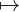Correcting functionsGeometric functionsAngle functionsExponential functionsAggregate functionsCalculation functions
StringString lengthLower / Upper casesConcatenateTrimPaddingOperationSubstringRegex
Date – Time – TimestampGet current DatetimeFormat DatetimeExtractionOperatorChecking
Other functions

### Numeric

#### Correcting functions

ABS(x): absolute of x.

CEIL(x) / CEILING(x): the smallest integer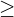x.

FLOOR(x): the largest integer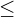x.

ROUND(x): the nearest integer to x.

ROUND(x, d): the nearest number with d decimal places.

#### Geometric functions

SIN(x): sin(x).

COS(x): cos(x).

TAN(x): tan(x).

COT(x): cot(x).

ACOS(x): arc-cos(x). x must be in the range [-1, 1] or else NULL is returned.

ASIN(x): arc-sin(x). x must be in the range [-1, 1] or else NULL is returned.

ATAN(x): arc-tan(x).

ATAN2(y, x): the angle between the ray to point (x, y) and the positive x-axis.(picture from wiki)
The output is confided in the range (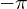,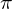).

#### Angle functions

DEGREES(x): convert x from radians to degrees.

PI(): the value of(3.14159…).

#### Exponential functions

EXP(x):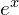.

LOG(x):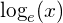.

LOG(b, x):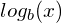.

POW(x, y) / POWER(x, y):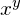.

SQRT(x):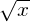.

#### Aggregate functions

GREATEST(x1, x2, x3, x4, …): return the biggest input.

LEAST(x1, x2, x3, x4, …): return the smallest input.

#### Calculation functions

MOD(n, m): n mod m

SIGN(x): return -1, 0 or 1 for negative, 0 and positive x, respectively.

### String

#### String length

BIT_LENGTH(s): length of s in bits.

CHAR_LENGTH(s) / CHARACTER_LENGTH(s): length of s in characters.

LENGTH(s): length of s in bytes.

#### Lower / Upper cases

LCASE(s) / LOWER(s): s in lowercase.

UCASE(s) / UPPER(s): s in uppercase.

#### Concatenate

CONCAT_WS(separator, s1, s2, s3, …): concatenate s1, s2, s3, etc. with separator.

CONCAT(s1, s2, s3, …): concatenate s1, s2, s3, etc. .

REPEAT(s, count): repeat s count times.

#### Trim

LTRIM(s): s with all spaces on the left removed.

RTRIM(s): s with all spaces on the right removed.

TRIM(s): s will all spaces at both ends removed.

TRIM([BOTH | LEADING | TRAILING] rem_s FROM s): trim all rem_s from s at both ends or on the left or on the right.

LPAD(s, len, s_pad): if the length of s is smaller than len, the function returns s with the string s_pad padded on the left until its length equal to len. If the length of s is bigger than len, return the first len characters of s.

RPAD(s, len, s_pad): if the length of s is smaller than len, the function returns s with the string s_pad padded on the right until its length equal to len. If the length of s is bigger than len, return the first len characters of s.

#### Operation

REPLACE(s, from_s, to_s): replace all from_s of s to to_s.

REVERSE(s): reverse s.

#### Substring

LEFT(s, len): the first len characters of s.

MID(s, pos, len) / SUBSTRING(s, pos, len) / SUBSTRING(s FROM pos FOR len): from the position pos of s, take len characters.

POSITION(substr IN s): the position of substr in s. (1-indexed.)

RIGHT(s, len): the last len characters of s.

SUBSTRING(s, pos) / SUBSTRING(s FROM pos): substring of s from pos position till the end.

#### Regex

REGEXP_MATCHES(s, p [, flag]): return any occurrences of POSIX pattern p in the string s.

REGEXP_REPLACE(s, p, to_s [, flag]): return s with POSIX pattern p replaced by to_s.

Some common regexp’s flags are:

• ‘i’ : case-insensitive.
• ‘g’: global search/replace.

### Date – Time – Timestamp

#### Get current Datetime

CURRENT_DATE: current date (at the start of the current transaction).

CURRENT_TIME: current time (at the start of the current transaction).

CURRENT_TIMESTAMP / NOW() / TRANSACTION_TIMESTAMP(): return current Datetime (at the start of the current transaction).

STATEMENT_TIMESTAMP(): return current Datetime (at the start of the current statement).

CLOCK_TIMESTAMP(): return current Datetime (may change during statement execution).

LOCALTIME: (at the start of the current transaction).

LOCALTIMESTAMP: (at the start of the current transaction).

TIMEOFDAY(): same as CLOCK_TIMESTAMP() but as a string.

#### Format Datetime

AGE(timestamp_1, timestamp_2): the interval between 2 timestamps (timestamp_1 – timestamp_2).

AGE(timestamp): the interval between the current time and the input timestamp.

DATE_TRUNC(field, timestamp): truncate the timestamp to the specified precision. Field.

JUSTIFY_DAYS(interval): adjust the interval so that 30-day is represented by a month.

JUSTIFY_HOURS(interval): adjust the interval so that 24-hour is represented by a day.

JUSTIFY_INTERVAL(interval): apply JUSTIFY_DAYS and JUSTIFY_HOURS.

TIMESTAMP WITHOUT TIME ZONE the_timestamp AT TIME ZONE zone: the_timestamp is understood to be in the default (UTC-7) time zone, then be adjusted to the new zone.

TIMESTAMP WITH TIME ZONE the_timestamp_with_zone AT TIME ZONE zone: the input timestamp is adjusted to the new zone.

TIME WITH TIME ZONE the_time_with_zone AT TIME ZONE zone: the input time is adjusted to the new zone.

TO_DATE(text, format): convert text into a date.

TO_TIMESTAMP(text, format): convert text into a timestamp.

#### Extraction

DATE_PART(‘field’, timestamp) / EXTRACT(field FROM timestamp): extract the field from the timestamp. Field.

DATE_PART(‘field’, interval) / EXTRACT(field FROM interval): extract the field from the interval. Field.

#### Operator

date – date: return an interval.

date +/- integer: return a date. the integer is understood to be days.

date +/- interval: return a timestamp.

date + time: return a timestamp.

timestamp +/- interval: return a timestamp.

timestamp – timestamp: return an interval.

interval +/- interval: return an interval.

interval * double: return an interval.

interval / double: return an interval.

time +/- interval: return a time.

time – time return an interval.

#### Checking

ISFINITE(date / timestamp / interval): return a boolean indicates if the input is finite.

Date field:

### Other functions

COALESCE(e1, e2, e3, …): return the first non-NULL value.

NULLIF(e1, e2): if e1 equals e2, NULL is returned, else e1 is returned.

CASE
WHEN condition_1 THEN value_1
WHEN condition_2 THEN value_2

[ELSE value_3]
END
: branching function.

References:

• Tutorialspoint’s Postgresql numeric functions: link